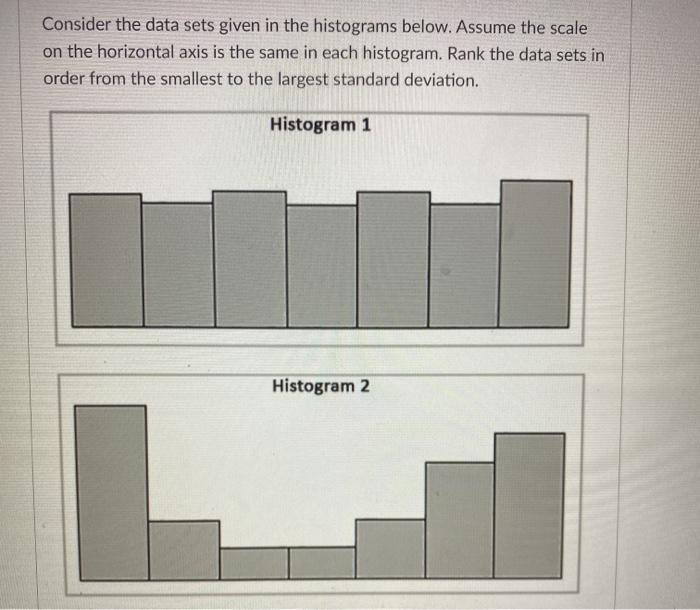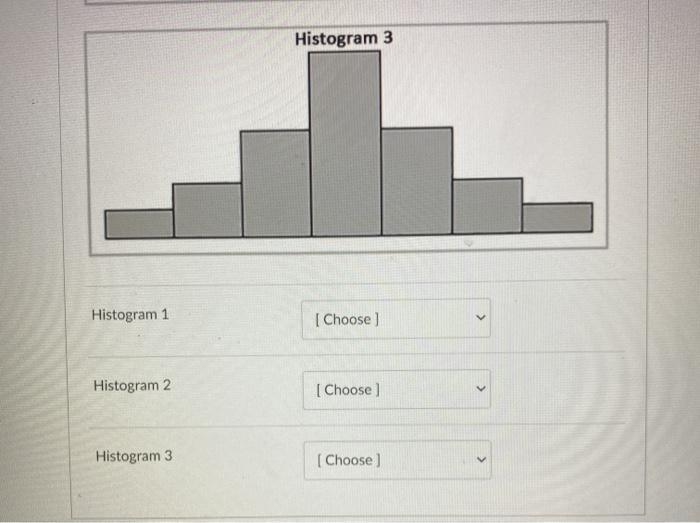# Question Solved1 AnswerConsider the data sets given in the histograms below. Assume the scale on the horizontal axis is the same in each histogram. Rank the data sets in order from the smallest to the largest standard deviation. Histogram 1 Histogram 2 Histogram 3 Histogram 1 [Choose) > Histogram 2 [ Choose < Histogram 3 [Choose) >IFHLJG The Asker · Probability and StatisticsTranscribed Image Text: Consider the data sets given in the histograms below. Assume the scale on the horizontal axis is the same in each histogram. Rank the data sets in order from the smallest to the largest standard deviation. Histogram 1 Histogram 2 Histogram 3 Histogram 1 [Choose) > Histogram 2 [ Choose < Histogram 3 [Choose) >
More
Transcribed Image Text: Consider the data sets given in the histograms below. Assume the scale on the horizontal axis is the same in each histogram. Rank the data sets in order from the smallest to the largest standard deviation. Histogram 1 Histogram 2 Histogram 3 Histogram 1 [Choose) > Histogram 2 [ Choose < Histogram 3 [Choose) >